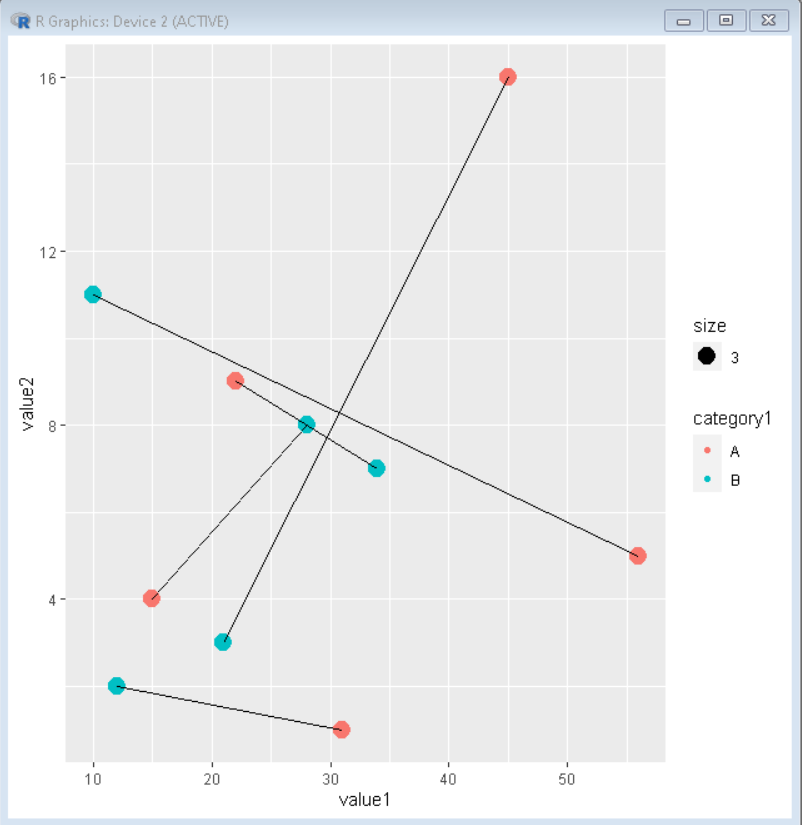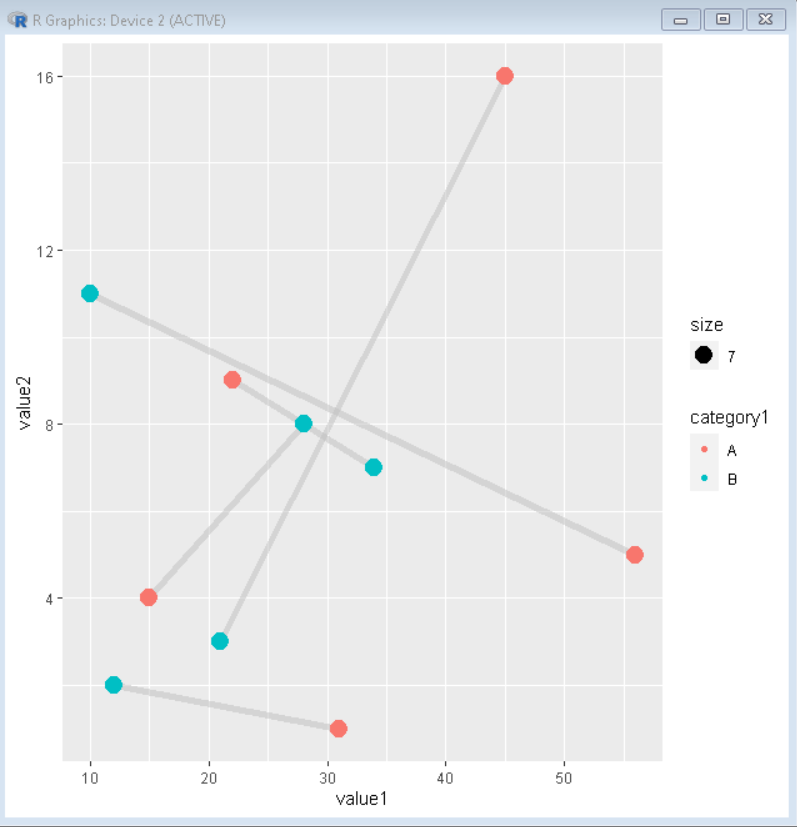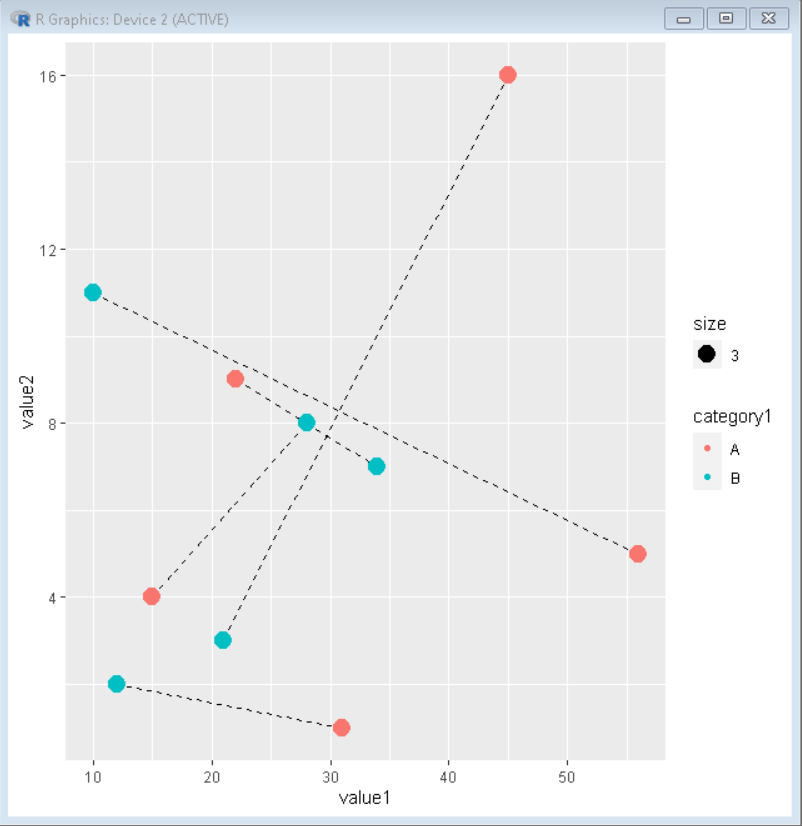Open in App
Not now

# How to Connect Paired Points with Lines in Scatterplot in ggplot2 in R?

• Last Updated : 05 Oct, 2021

In this article, we will discuss how to connect paired points in scatter plot in ggplot2 in R Programming Language.

Scatter plots help us to visualize the change in two more categorical clusters of data. Sometimes, we need to work with paired quantitative variables and try to visualize their relationship. For example, we may have two quantitative variables corresponding to two different categories and would like to connect those data points by lines.

Syntax: ggplot(aes( x, y )) + geom_point( aes( color ) ) + geom_line( aes( group ) )

Where:

• group: the variable that has pairs to be joined.
• color: the variable that categorizes points

### Example 1:  Creating a Scatter plot with lines joining paired points

Here is a basic scatter plot with lines joining paired points.

## R

 `# create dataframe``sample_data <- ``data.frame``( value1 = ``c``(31,12,15,28,45,``                                      ``21,22,34,56,10),``                           ` `                          ``value2 = ``c``(1,2,4,8,16,3,9,``                                     ``7,5,11),``                           ` `                          ``category1 = ``c``(``'A'``,``'B'``,``'A'``,``'B'``,``'A'``,``                                        ``'B'``,``'A'``,``'B'``,``'A'``,``'B'``),``                           ` `                          ``category2 = ``c``(0,0,1,1,2,2,3,3,4,4))``# load tidyverse``library``(tidyverse)`` ` `# create plot``ggplot``(sample_data, ``aes``(value1,value2)) +``  ``geom_point``(``aes``(color=category1, size=3)) +``  ``geom_line``(``aes``(group = category2))`

Output:## Color Customization

We can change the color and width of the joining segment by using the color and size property of geom_line().

## R

 `# create dataframe``sample_data < - ``data.frame``(value1=``c``(31, 12, 15, 28, 45, 21,``                                    ``22, 34, 56, 10),``                           ``value2=``c``(1, 2, 4, 8, 16, 3, 9, 7, 5, 11),`` ` `                           ``category1=``c``(``'A'``, ``'B'``, ``'A'``, ``'B'``, ``'A'``,``                                       ``'B'``, ``'A'``, ``'B'``, ``'A'``, ``'B'``),`` ` `                           ``category2=``c``(0, 0, 1, 1, 2, 2, 3, 3, 4, 4))``# load tidyverse``library``(tidyverse)`` ` `# create plot``ggplot``(sample_data, ``aes``(value1, value2)) +``geom_line``(``aes``(group=category2), color=``"grey"``,``          ``size=2, alpha=0.5) +``geom_point``(``aes``(color=category1, size=7))`

Output:## Line Customization

We can convert the joining line into ridged line by using linetype property. Every property that works on a line plot also works in this line.

## R

 `# create dataframe``sample_data < - ``data.frame``(value1=``c``(31, 12, 15, 28, 45, 21,``                                    ``22, 34, 56, 10),`` ` `                           ``value2=``c``(1, 2, 4, 8, 16, 3, 9, 7, 5, 11),`` ` `                           ``category1=``c``(``'A'``, ``'B'``, ``'A'``, ``'B'``, ``'A'``, ``'B'``,``                                       ``'A'``, ``'B'``, ``'A'``, ``'B'``),`` ` `                           ``category2=``c``(0, 0, 1, 1, 2, 2, 3, 3, 4, 4))``# load tidyverse``library``(tidyverse)`` ` `# create plot with ridged line``ggplot``(sample_data, ``aes``(value1, value2)) +``geom_line``(``aes``(group=category2), linetype=2) +``geom_point``(``aes``(color=category1, size=3))`

Output:My Personal Notes arrow_drop_up